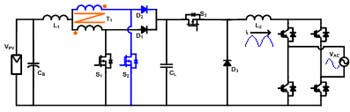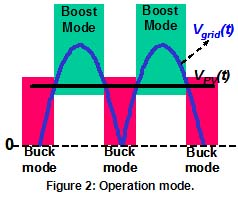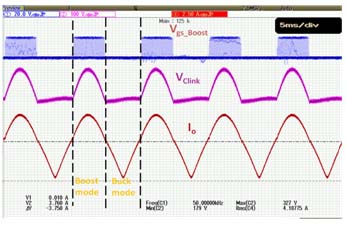LIBRARY

# Derivation of High Efficient Robust PV InverterFig. 1. Proposed interleaved boost plus buck PV inverter.
Photovoltaic power supplied to the utility grid is gaining more and more attention. Depending on the characteristics of PV panels, the total output voltage from the PV panels varies a lot due to different temperature and irradiation conditions and the shading and clouding effects. Thus, the input voltage of a residential PV inverter can vary in a wide range, for example from 200 V to 500 V, which appears to be highly mismatched with the desirable 400-V level. Therefore, a dc-dc converter with either step-up or step-down function or even both step-up and -down functions is needed before the dc-ac inverter stage. Such a dc-dc converter in conjunction with a dc-ac inverter arrangement is considered the state-of-the-art PV PCS.

An interleaved boost-buck dc-dc converter is proposed as the first stage with regulated output inductor current, and a full-bridge unfolding circuit with 50- or 60-Hz line frequency is applied to the dc-ac sage, which will unfold the rectified sinusoid current regulated by the dc-ac stage into a pure sinusoidal current, as shown in Figure 1. The buck mode will work if the input voltage is higher than the instant grid voltage, otherwise the boost mode will work. These two modes work separately as shown in Figure 2. After modeling of the inverter, it is known that the smaller L1 is the better controller can be designed and the better performance can be obtained. Thus, interleaved boost is proposed in order to have the same current ripple. The experimental results are shown in Figure 3 (a) and (b).Fig. 2. Operation mode.Fig. 3. (a) Experiment Results of PWM Signals.Fig. 3. (b) Experiment Results.